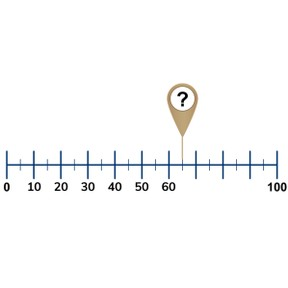Number line to 100 by 5s and 10s

# Number line to 100 by 5s and 10s

No account needed.8,000 schools use Gynzy92,000 teachers use Gynzy1,600,000 students use Gynzy

## General

Students learn to place 5s and 10s on the number line to 100. They learn that long marks on a number line show tens numbers and short marks show fives numbers.

-1.NBT.B.2

## Relevance

Discuss with students that it is important to be able to place a number on the number line so you know where a number belongs. You can use this to determine when a number is bigger or smaller than the given number.

## Introduction

Practice recognizing numbers that end on 0 and 5 (the tens and fives). Ask students to say the numbers and drag them to the correct boxes.

## Development

Explain what a number line is to your students. Show students that a longer mark represents a tens number and a shorter mark represents a fives number. Explain tens and fives to your students and that you skip count in 5s or 10s from left to right. Practice counting with the students. Together with the class, determine which numbers belong at the arrows. Tell students to first put tens on a number line, and then fives. Also tell them that they should skip count in 10s first, and then move to 5s.

Check that students understand placing numbers to 100 on the number line in 10s and 5s by asking the following questions:
- What is a number line?
- How do you place a number on the number line?
- Where do you start counting?
- Which jumps can you take on a number line?

## Guided practice

Students practice placing numbers on the number line. They first get to select from four options, and later need to choose the answers themselves.

## Closing

Discuss with students that it is important to be able to place numbers on the number line because you then know where it belongs. You can also use the longer mark to find a tens number, or a shorter mark to find the fives number. Have students practice in pairs. Have each student draw a number line and write 3 tens numbers and 3 fives numbers below the line. Trade papers with your partner and write the given numbers on the correct places on the number lines.

## Teaching tips

Students who have difficulty placing 10s and 5s on the number line can practice skip counting to 100 in 5s, and in 10s.

### The online teaching platform for interactive whiteboards and displays in schools

• Save time building lessons

• Manage the classroom more efficiently

• Increase student engagement